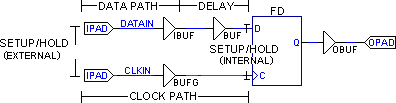# AR# 13309

|

## 描述

Why does the setup/hold window that is specified in the data sheet, TRCE, and Timing Analyzer increase in size when I use the delay element? The window should just move toward more setup with the addition of delay on the input data path.

## 解决方案

Adding pure delay will not change the size of a data valid window. The addition of delay that has maximum and minimum values associated with it will always increase the window by the difference between the maximum and minimum values of the added element.

Consider the following diagram:External I/O Circuit

Suppose that you begin at an internal flip-flop that has a window of 1 ns (setup)/0 ns (hold) (internal), which creates a 1 ns window. Note that this window is at the pins of the internal flip-flop itself, deep inside the IOB or CLB.

Now, examine the window at the pins for a case without the delay element. Suppose that the data path has a worst-case delay of 2 ns and a best-case delay of 1.5 ns. Assume that the clock path has a worst-case delay of 0.5 ns and a best-case delay of 0 ns.

So, the calculations for external setup/hold will be (without delay):

Setup = (internal)setup + (worst) data - (best) clock = 1 ns + 2 ns - 0 ns = 3 ns setup
Hold = (internal)hold - (best) data + (worst) clock = 0 ns - 1.5 ns + 0.5 ns = -1 ns hold

Therefore, the window is 3 + -1= 2 ns. Tracing out to the pins increased the window by 1 ns.

Now, include the delay element, which adds at least 2.5 ns (at most 3 ns) of delay to the data line.

The new calculations are (with delay):

Setup = (internal)setup + (worst) data + (worst) delay element - (best)clock = 1 ns + 2 ns + 3 ns - 0 ns = 6 ns setup
Hold = (internal)hold - (best) data - (best) delay element + (worst)clock = 0 ns - 1.5 ns -2.5 ns +.5 ns = -3.5 ns hold

The window is now 6 + -3.5 ns = 2.5 ns. This is 0.5 ns larger than without the delay element. Notice that the change in the window is equal to the worst-case -to- best-case numbers for the delay element.

This can be proven in another way:

Start with the equation for the window size, then substitute the equations for Setup and Hold. Next, consolidate the terms, where "d" is the difference between worst-case and best-case values for that particular parameter:

window = biggest setup time + biggest hold time = (internal)setup + (worst) data + (worst) delay element - (best) clock + (internal)hold - (best) data - (best) delay element + (worst) clock = (internal)setup +(internal)hold + ddata +dclock +ddelay

So, dwindow = window with delay - window without delay = (internal)setup +(internal)hold + ddata +dclock +ddelay - ((internal)setup +(internal)hold + ddata +dclock) = ddelay

You can now see that all of the numbers are positive. If additional "ddelay" (which has its own best/worst timing parameters) is added, the window will always increase. The only way for a window to shrink would be if negative delay existed, meaning that the worst-case timing is faster than the best-case. This is not possible.

AR# 13309

Type 已知问题
Tools
People Also Viewed